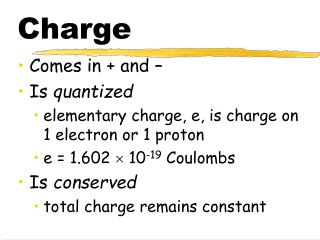DownloadDownload PresentationCharge

# Charge

Télécharger la présentation## Charge

- - - - - - - - - - - - - - - - - - - - - - - - - - - E N D - - - - - - - - - - - - - - - - - - - - - - - - - - -
##### Presentation Transcript

1. Charge • Comes in + and – • Is quantized • elementary charge, e, is charge on 1 electron or 1 proton • e = 1.602  10-19 Coulombs • Is conserved • total charge remains constant

2. Coulomb’s Law • F = kq1q2/r2 • k = 8.99  109 N m2 / C2 • q1, q2 are charges (C) • r2 is distance between the charges (m) • F is force (N) • Applies directly to spherically symmetric charges

3. F =kqq0 r2 E = F = kq q0 r2 Spherical Electric Fields force field

4. Why use fields? • Forces exist only when two or more particles are present. • Fields exist even if no force is present. • The field of one particle only can be calculated.

5. Field around + charge Positive charges accelerate in direction of lines of force Negative charges accelerate in opposite direction

6. Field around - charge Positive charges follow lines of force Negative charges go in opposite direction

7. For any electric field • F = Eq • F: Force in N • E: Field in N/C • q: Charge in C

8. Principle of Superposition When more than one charge contributes to the electric field, the resultant electric field is the vector sum of the electric fields produced by the various charges.

9. Field around dipole

10. Caution… Electric field lines are NOT VECTORS, but may be used to derive the direction of electric field vectors at given points. The resulting vector gives the direction of the electric force on a positive charge placed in the field.

11. Field Vectors

12. U = kqq0 r V = U = kq q0 r Electric Potential potential energy potential (for spherically symmetric charges)

13. Electrical Potential V = -Ed V:change in electrical potential (V) E: Constant electric field strength (N/m or V/m) d:distance moved (m)

14. Electrical Potential Energy U = qV U: change in electrical potential energy (J) q: charge moved (C) V: potential difference (V)

15. Electrical Potentialand Potential Energy Are scalars!

16. Potential Difference • Positive charges like to DECREASE their potential. • (DV < 0) • Negative charges like to INCREASE their potential. • (DV > 0)

17. highest high medium low lowest Potential surfaces positive negative

18. Equipotential surfaces high low

19. Definition: Capacitor • Consists of two “plates” in close proximity. • When “charged”, there is a voltage across the plates, and they bear equal and opposite charges. • Stores electrical energy.

20. Capacitance C = q / DV C: capacitance in Farads (F) q: charge (on positive plate) in Coulombs (C) V: potential difference between plates in Volts (V)

21. Energy in a Capacitor UE = ½ C (DV)2 U: electrical potential energy (J) C: capacitance in (F) V: potential difference between plates (V)

22. Capacitance of parallel plate capacitor C = ke0A/d C: capacitance (F) ke: dielectric constant of filling 0 : permittivity (8.85 x 10-12 F/m) A: plate area (m2) d: distance between plates(m)

23. +Q V1 V2 E V3 V4 V5 -Q Parallel Plate Capacitor dielectric

24. - Q + Q E Cylindrical Capacitor

25. Problem #1 Calculate the force on the 4.0 C charge due to the other two charges. +4 C 60o 60o +1 C +1 C

26. Problem #2 Calculate the mass of ball B, which is suspended in midair. q = 1.50 nC A R = 1.3 m q = -0.50 nC B

27. Problem #3 Two 5.0 C positive point charges are 1.0 m apart. What is the magnitude and direction of the electric field at a point halfway between them?

28. Problem #4 Calculate the magnitude of the charge on each ball, presuming they are equally charged. 40o 1.0 m 1.0 m A B 0.10 kg 0.10 kg